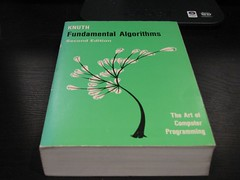# Print numbers from 1 to n using loops, recursion or goto statement

You may have made program to print numbers from 1 to n using loops, recursion or goto statement. But have you ever thought about doing this without help of loops, recursion or goto? It can be done without using such things as explained in below program.

#include<iostream>
using namespace std;
class Num
{
public:
static int i;
Num()
{
cout<<i++<<” “;
}
};
int Num::i=1;
int main()
{
int n;
cout<<“Enter value on n:”;
cin>>n;
Num obj[n];
return 0;
}
In this program we are using the concept of static data member and array of objects. Class Numcontains a static variable i whose value will remain till the program terminates. We are creating an arrayof objects of class Num. In this program we are creating n objects, value of n depends on input we give. The default constructor is called for all objects one by one. In the constructor the value of i is printed and is incremented by one in each call. In this way numbers from 1 to n are printed.

# Array Descending Order

`Problem: Array Ascending Order `
#include<stdio.h>

#include<conio.h>

void main(void)

{

clrscr();

int i,j,c,num,a;

printf(“\nProg: Ascending Order”);

printf(“\nEnter How many Number:..”);

scanf(“%d”,&num);

printf(“\nEnter Integer:”);

for(i=0;i

{

scanf(“%d”,&a[i]);   /* input data */

}

for(i=0;i

{

for(j=i+1;j<num;j++)

{

if(a[i]>a[j])

{

c=a[i];

a[i]=a[j];

a[j]=c;

}

}

}

printf(“\nAscending Order:”);   /* Sorting Ascending Order */

for(i=0;i<num;i++)

{

printf(“\n%d”,a[i]);

}

getch();

}

# Problem 04 | ArrayClass function

`Problem : Take 10 Number as input, print 5th Number in another function`

#include<stdio.h>

#include<conio.h>

#define n 10

void sum(int a);

/* prototype function */   void main(void)

{

clrscr();

int i,A[n];

printf(“\nEnter Number:…”);

for(i=1;i

sum(A);        /*call function & print 5th number */

getch();

}

void sum(int a)

{

printf(“\nResult:%d”,a);

}

# Primary and Basic knowledge of C |The Art of Computer Programming, Volume 1: Fundamental Algorithms (Photo credit: mrbill)

C is the basic level of the computer programming. Anyone wants to be good software engineers, then he or she must should be good knowledge on the base level of the computer programming. So, of course, you should be a better knowledge and programming experience on C, C++.

Why : ” C “ is the base level for the begging of the programming. You can achieve the coding  style, input methodology, cycling, looping, execution, developing everything step by step from this ” C ‘ language. For the better programming you must should be a good knowledge on data type, variable, memory location, operator, looping, function, recursion, structure and much more. Here, I mentioned some basic data type, which is very important for programming in C, C+.

Data types are different but basically there are 6 types of data use for C’ programming, such as Integer, float, long, double, Char, Char [ ].# Problem 03

/* 1^2+2+3+………+100  */